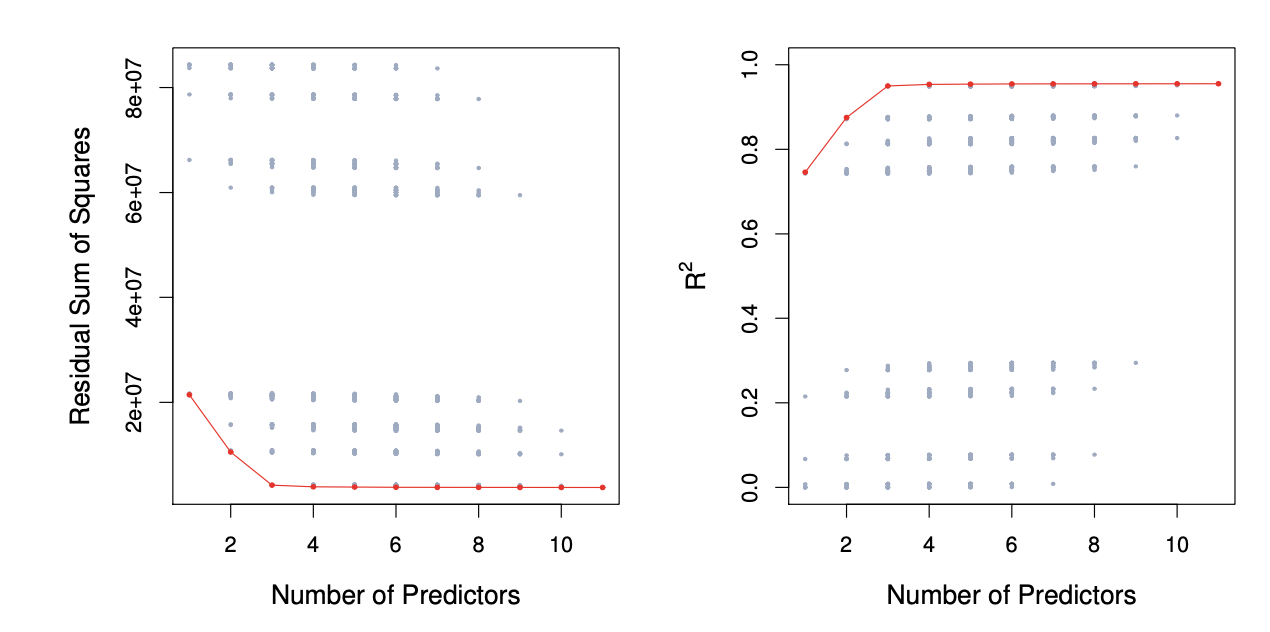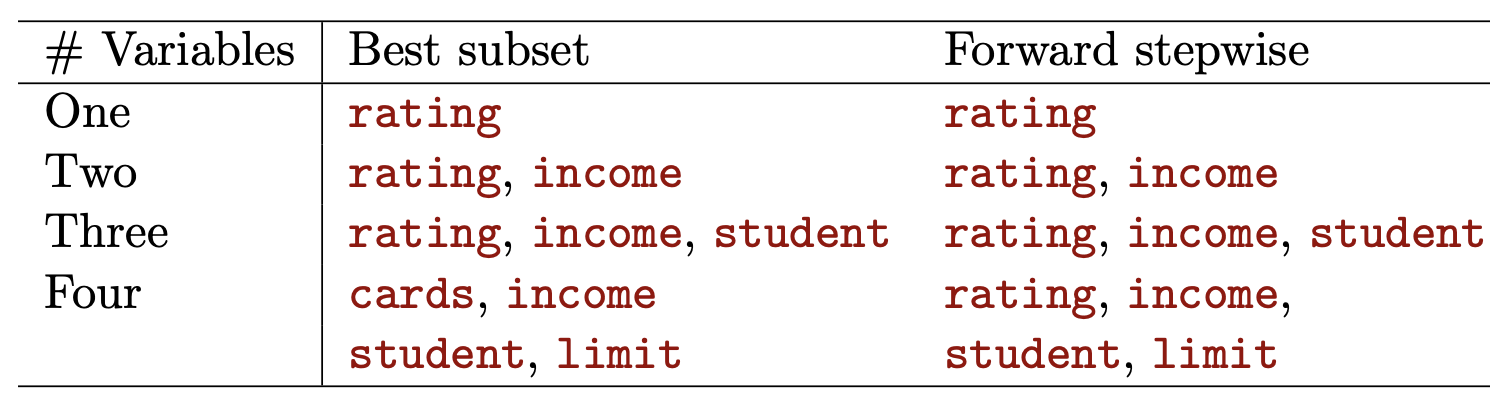## Linear Model Selection and Regularization

• Recall the linear model:

• $$Y = B_0 + B_1X_1 + ... + B_pX_p + \epsilon$$
• We will consider some approaches for extending the linear model framework. In Chapter 7, we will examine generalizations of the linear model in order to accommodate non-linear, but still additive, relationships

• In Chapter 8 we consider even more general non-linear models

• Despite its simplicity, the linear model has distinct advantages in terms of its interpretability and often shows good predictive performance

• Hence we discuss some ways in which the simple linear model can be improved, by replacing ordinary least squares fitting with some alternative fitting procedures

### Why Consider Alternatives to Least Squares?

• Prediction Accuracy:

• especially when \$p > n4, to control the variance
• Model Interpretability:

• By removing irrelevant features — that is, by setting the corresponding coefficient estimates to zero — we can obtain a model that is more easily interpreted

• We will present some approaches for automatically performing feature selection

## Three Classes of Methods

• Subset Selection:

• We identify a subset of the $$p$$ predictors that we believe to be related to the response

• We then fit a model using least squares on the reduced set of variables

• Shrinkage:

• We fit a model involving all $$p$$ predictors, but the estimated coefficients are shrunken towards zero relative to the least squares estimates

• This shrinkage (also known as regularization) has the effect of reducing variance and can also perform variable selection

• Dimension Reduction:

• We project the $$p$$ predictors into a M-dimensional subspace, where $$M < p$$

• This is achieved by computing $$M$$ different linear combinations, or projections, of the variables

• Then these M projections are used as predictors to fit a linear regression model by least squares

### Subset Selection

• Best Subset Selection Procedures

• Let $$M_0$$ denote the null model, which contains no predictors. This model simply predicts the sample mean for each observation

• For $$k = 1,2,...p$$

• Fit all $$\binom{p}{k}$$ models that contain exactly $$k$$ predictors

• Pick the best among these $$\binom{p}{k}$$ models and call it $$M_k$$

• Where best is defined as having the smallest RSS or equivalently largest $$R^2$$
• Select a single best model from among $$M_0,...,M_p$$ using cross-validation prediction error, $$C_p$$ (AIC), BIC, or adjusted $$R^2$$

• Example: Credit Data Set• For each possible model containing a subset of the ten predictors in the Credit data set, the RSS and $$R_2$$ are displayed. The red frontier tracks the best model for a given number of predictors, according to RSS and $$R_2$$. Though the data set contains only ten predictors, the x-axis ranges from 1 to 11, since one of the variables is categorical and takes on three values, leading to the creation of two dummy variables

### Extensions to Other Models

• Although we have presented best subset selection here for least squares regression, the same ideas apply to other types of models, such as logistic regression

• The deviance— negative two times the maximized log-likelihood— plays the role of RSS for a broader class of models

## Stepwise Selection

• For computational reasons, best subset selection cannot be applied with very large $$p$$

• Best subset selection may also suffer from statistical problems when $$p$$ is large: larger the search space, the higher the chance of finding models that look good on the training data, even though they might not have any predictive power on future data

• Thus an enormous search space can lead to overfitting and high variance of the coefficient estimates

• For both of these reasons, stepwise methods, which explore a far more restricted set of models, are attractive alternatives to best subset selection

### Forward Stepwise Selection

• Forward stepwise selection begins with a model containing no predictors, and then adds predictors to the model, one-at-a-time, until all of the predictors are in the model

• In particular, at each step the variable that gives the greatest additional improvement to the fit is added to the model

• Computational advantage over best subset selection is clear

• It is not guaranteed to find the best possible model out of all $$2^p$$ models containing subsets of the $$p$$ predictors

• Forward Stepwise Selection

• Let $$M_0$$ denote the null model, which contains no predictors

• For $$k = 0,...,p-1$$:

• Consider all $$p-k$$ models that augment the predictors in $$M_k$$ with one additional predictor

• Choose the best among these $$p-k$$ models, and call it $$M_{k+1}$$. Here best is defined as having the smallest RSS or highest $$R_2$$

• Select a single best model from among $$M_0,...,M_p$$ using cross-validated prediction error, $$C_p$$ (AIC), BIC, or adjusted $$R^2$$

• Example: Credit Data• The first four selected models for best subset selection and forward stepwise selection on the Credit data set. The first three models are identical but the fourth models differ

## Backward Stepwise Selection

• Like forward stepwise selection, backward stepwise selection provides an efficient alternative to best subset selection

• However, unlike forward stepwise selection, it begins with the full least squares model containing all $$p$$ predictors, and then iteratively removes the least useful predictor, one-at-a-time

• Like forward stepwise selection, the backward selection approach searches through only $$1 + p(p + 1)/2$$ models, and so can be applied in settings where p is too large to apply best subset selection

• Like forward stepwise selection, backward stepwise selection is not guaranteed to yield the best model containing a subset of the $$p$$ predictors

• Backward selection requires that the number of samples n is larger than the number of variables $$p$$ (so that the full model can be fit). In contrast, forward stepwise can be used even when $$n < p$$, and so is the only viable subset method when $$p$$ is very large

• Backward Stepwise Selection

• Let $$M_p$$ denote the full model, which contains all $$p$$ predictors

• For $$k=p, p-1,...,1$$:

• Consider all $$k$$ models that contain all but one of the predictors in $$M_k$$ for a total of $$k-1$$ predictors

• Choose the best among these $$k$$ models, and call it $$M_{k-1}$$. Here best is defined as having the smallest RSS or highest $$R^2$$

• Select a single best model from among $$M_0,...,M_p$$ using cross-validation prediction error, $$C_p$$ (AIC), BIC, or adjusted $$R^2$$

## Choosing the Optimal Model

• The model containing all of the predictors will always have the smallest RSS and the largest $$R^2$$, since these quantities are related to the training error

• We wish to choose a model with low test error, not a model with low training error. Recall that training error is usually a poor estimate of test error

• Therefore, RSS and $$R^2$$ are not suitable for selecting the best model among a collection of models with different numbers of predictors

## Estimating Test Error: Two Approaches

• We can indirectly estimate test error by making an adjustment to the training error to account for the bias due to overfitting

• We can directly estimate the test error, using either a validation set approach or a cross-validation approach

### $$C_p$$, AIC, BIC< and Adjusted $$R^2$$

• These techniques adjust the training error for the model size, and can be used to select among a set of models with different numbers of variables

• The figure below displays $$C_p$$, BIC, and adjusted $$R^2$$ for the best model of each size produced by the best subset selection on the Credit data set